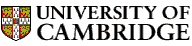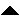Computer LaboratoryComputer Science Syllabus - Logic and ProofLogic and ProofNext: Mathematical Methods for Computer Up: Michaelmas Term 2005: Part Previous: Group Project   Contents

## Logic and Proof

Lecturer: Professor L.C. Paulson

No. of lectures: 12

This course is a prerequisite for Artificial Intelligence II (Part II) and Specification and Verification I (Part II).

Aims

This course will teach logic, especially the predicate calculus. It will present the basic principles and definitions, then describe a variety of different formalisms and algorithms that can be used to solve problems in logic. Putting logic into the context of Computer Science, the course will show how the programming language Prolog arises from the automatic proof method known as resolution. It will introduce topics that are important in mechanical verification, such as binary decision diagrams (BDDs) and modal logic.

Lectures

• Introduction to logic. Schematic statements. Interpretations and validity. Logical consequence. Inference.

• Propositional logic. Basic syntax and semantics. Equivalences. Normal forms. Tautology checking using CNF.

• The sequent calculus. A simple (Hilbert-style) proof system. Natural deduction systems. Sequent calculus rules. Sample proofs.

• Binary decision diagrams. General concepts. Fast canonical form algorithm. Optimisations. Applications.

• First order logic. Basic syntax. Quantifiers. Semantics (truth definition).

• Formal reasoning in FOL. Free versus bound variables. Substitution. Equivalences for quantifiers. Sequent calculus rules. Examples.

• Clausal proof methods. Clause form. The Davis-Putnam procedure. The resolution rule. Examples. Refinements.

• Skolem functions and Herbrand's theorem. Prenex normal form. Skolemisation. Herbrand models and their properties.

• Unification. Composition of substitutions. Most general unifiers. A unification algorithm. Applications and variations.

• Prolog. Binary resolution. Factorisation. Example of Prolog execution. Proof by model elimination.

• Modal logics. Possible worlds semantics. Truth and validity. A Hilbert-style proof system. Sequent calculus rules.

• Tableaux methods. Simplifying the sequent calculus. Examples. Adding unification. Skolemisation. The world's smallest theorem prover?

Objectives

At the end of the course students should

• be able to manipulate logical formulas accurately

• be able to perform proofs using the presented formal calculi

• be able to construct a small BDD

• understand the relationships among the various calculi, e.g. Davis-Putnam, resolution and Prolog

• be able to apply the unification algorithm and to describe its uses

* Huth, M. & Ryan, M. (2004). Logic in computer science: modelling and reasoning about systems. Cambridge University Press (2nd ed.).
Ben-Ari, M. (2001). Mathematical logic for computer science. Springer (2nd ed.).

Gentle introduction:
Barwise, J. & Etchemendy, J. (1999). Language proof and logic. CSLI Publications.Next: Mathematical Methods for Computer Up: Michaelmas Term 2005: Part Previous: Group Project   Contents
Christine Northeast
Sun Sep 11 15:46:50 BST 2005© 2005 University of Cambridge Computer Laboratory Please send any comments to pagemaster@cl.cam.ac.uk Page last updated on 11-Sep-2005 at 15:57 by Christine Northeast